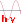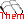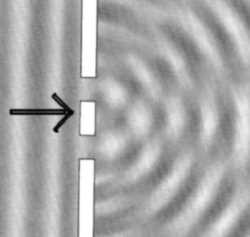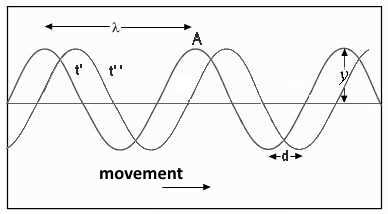Waves

At the water surface, a wave approaches a wall with a double slit. Behind the wall is observed the interference phenomenon. It is observed that on a line parallel to the wall, the maxima and minima are alternated regularly.A beam of light from a laser is expanded means of a glass rod, and then projected on a wall provided with a double slot. A screen placed behind the wall shows alternate light and dark bands: This phenomenon is similar to the interference that described above.Light is a wave phenomenonThe wave represented moves from left to right. It is represented at time t'and t'' with t''> t '. During the time t = t''- t', it has traveled the distance d. We define:

Speed of the wave (unit: $1\;\frac{m}{s}$): $v$ $=$ $\frac{d}{t}\;(1)$ Wavelength (unit: $1\; m$): $\lambda$ = distance between two consecutive peaks in a given time

An observer at A sees the top of the wave scrolling along. After time $T$, he sees the next cycle peak. The wave then covered the distance $\lambda$. We define:

Period of the wave (unit: $1\;s$): $T$ = time it takes for a point on the wave to travel the distance $\lambda$

Using (1), the fundamental relationship is obtained:

Fundamental relationship: $v$ $=$ $\frac{\lambda}{T}\;(2)$

An observer at A sees scrolling by $\nu$ peaks of the wave in one second, ie the time interval $T$ is included $\nu$ times in a second. We define:

Frequency wave} (unit: 1 Hertz = $1 Hz$ $=$ $1s^{- 1})$: $\nu$ $=$ $\frac{1}{T}\;(3)$

$\nu$ is the number of times a point of the wave travels distance $\lambda$ in a second. Using (2) and (3), we reformulate the fundamental relationship:

$v$ $=$ $\lambda\cdot\nu\; (4)$